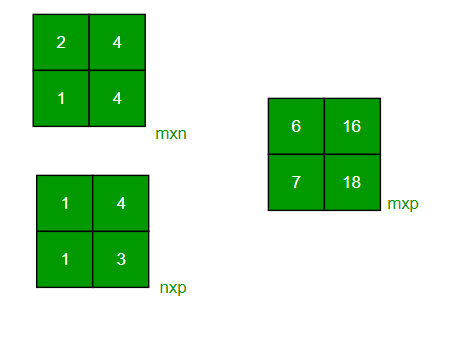# Java Program to Multiply two Matrices of any size

• Difficulty Level : Basic
• Last Updated : 01 Sep, 2021

Given two matrices A and B of any size, the task to multiply them in Java.
Examples:

```Input: A[][] = {{1, 2},
{3, 4}}
B[][] = {{1, 1},
{1, 1}}
Output: {{3, 3},
{7, 7}}

Input: A[][] = {{2, 4},
{3, 4}}
B[][] = {{1, 2},
{1, 3}}
Output: {{6, 16},
{7, 18}}```

Attention reader! All those who say programming isn't for kids, just haven't met the right mentors yet. Join the  Demo Class for First Step to Coding Coursespecifically designed for students of class 8 to 12.

The students will get to learn more about the world of programming in these free classes which will definitely help them in making a wise career choice in the future.

Approach:

• Take the two matrices to be multiplied
• Check if the two matrices are compatible to be multiplied• Create a new Matrix to store the product of the two matrices
• Traverse each element of the two matrices and multiply them. Store this product in the new matrix at the corresponding index.
• Print the final product matrix

Below is the implementation of the above approach:

## Java

 `// Java program to multiply two square matrices.` `import` `java.io.*;` `class` `GFG {` `    ``// Function to print Matrix``    ``static` `void` `printMatrix(``int` `M[][],``                            ``int` `rowSize,``                            ``int` `colSize)``    ``{``        ``for` `(``int` `i = ``0``; i < rowSize; i++) {``            ``for` `(``int` `j = ``0``; j < colSize; j++)``                ``System.out.print(M[i][j] + ``" "``);` `            ``System.out.println();``        ``}``    ``}` `    ``// Function to multiply``    ``// two matrices A[][] and B[][]``    ``static` `void` `multiplyMatrix(``        ``int` `row1, ``int` `col1, ``int` `A[][],``        ``int` `row2, ``int` `col2, ``int` `B[][])``    ``{``        ``int` `i, j, k;` `        ``// Print the matrices A and B``        ``System.out.println(``"\nMatrix A:"``);``        ``printMatrix(A, row1, col1);``        ``System.out.println(``"\nMatrix B:"``);``        ``printMatrix(B, row2, col2);` `        ``// Check if multiplication is Possible``        ``if` `(row2 != col1) {` `            ``System.out.println(``                ``"\nMultiplication Not Possible"``);``            ``return``;``        ``}` `        ``// Matrix to store the result``        ``// The product matrix will``        ``// be of size row1 x col2``        ``int` `C[][] = ``new` `int``[row1][col2];` `        ``// Multiply the two matrices``        ``for` `(i = ``0``; i < row1; i++) {``            ``for` `(j = ``0``; j < col2; j++) {``                ``for` `(k = ``0``; k < row2; k++)``                    ``C[i][j] += A[i][k] * B[k][j];``            ``}``        ``}` `        ``// Print the result``        ``System.out.println(``"\nResultant Matrix:"``);``        ``printMatrix(C, row1, col2);``    ``}` `    ``// Driver code``    ``public` `static` `void` `main(String[] args)``    ``{` `        ``int` `row1 = ``4``, col1 = ``3``, row2 = ``3``, col2 = ``4``;` `        ``int` `A[][] = { { ``1``, ``1``, ``1` `},``                      ``{ ``2``, ``2``, ``2` `},``                      ``{ ``3``, ``3``, ``3` `},``                      ``{ ``4``, ``4``, ``4` `} };` `        ``int` `B[][] = { { ``1``, ``1``, ``1``, ``1` `},``                      ``{ ``2``, ``2``, ``2``, ``2` `},``                      ``{ ``3``, ``3``, ``3``, ``3` `} };` `        ``multiplyMatrix(row1, col1, A,``                       ``row2, col2, B);``    ``}``}`
Output:
```Matrix A:
1 1 1
2 2 2
3 3 3
4 4 4

Matrix B:
1 1 1 1
2 2 2 2
3 3 3 3

Resultant Matrix:
6 6 6 6
12 12 12 12
18 18 18 18
24 24 24 24```

My Personal Notes arrow_drop_up